# How To Estimate Optimum Insulation Thickness

In previous post I shared how to estimate insulation thickness for both flat surface and cylindrical surface. In this post I want to share how to estimate optimum insulation thickness.

The total annual cost of insulation is the sum of the cost of heat energy lost and fixed cost. A plot of cost vs insulation thickness will determine the most economical insulation thickness. A dimensionless factor can be used to calculate insulation thickness, depending on the ratio of insulation thickness to pipe diameter in the following equation:

Where:

t              = operating time in hours per year

Ch            = annual cost of heat loss (\$/million Btu)

Ci             = installed cost of insulation per cubic foot

f              = fraction of installed cost depreciated annually

D             = outside diameter of pipe (in)

K             = thermal conductivity of insulation material (Btu/hr.ft.oF)

∆to          = difference between surface temperature and ambient air temperature (F)

h             = combined film coefficient of convection and radiation in the air film on the insulator (Btu/hr.ft2.F)

To get h, we used the following Equation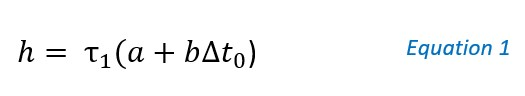Where:

τ1            = dimensionless factor depending on the surface (see Table 1)

τ2            = dimensionless factor (see Chart 1)

a              = 1.2 Btu/ft2.F.hr

b             = 0.0048 Btu/ft2.F.hr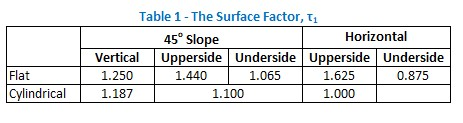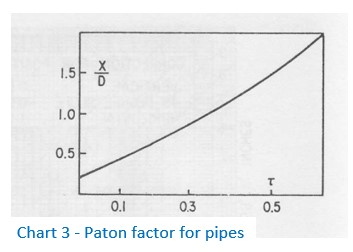The unknown surface temperature can be found in parallel with optimum insulation thickness by trial and error. The approach used to find optimum insulation thickness is to plot ∆to vs X (insulation thickness) from figure below and ∆to vs X (insulation thickness) from equation above and find the point of intersection.

Procedures

The recommended procedures to find optimum insulation thickness is as follows:

1. Make a rough guess at the surface temperature and select two other points, say 25 degrees above and below this value.
2. Find the corresponding insulation thickness for each of the three points by two ways: first using chart 1, chart 2, and chart 3 above and second using Equation 1.
3. Plot these points with ∆to for the X-axis and the insulation thickness for the Y-axis and connect them with two smooth curves. The intersection of the two curves indicates the optimum thickness.

Example

Let say we have vertical pipe with the following data.

Outside diameter             : 4 in

Air temperature               : 85 F

Fluid temperature           : 650 F

Thermal conductivity of insulation material          : 0.05 Btu/hr.ft.F (at 400 F)

Estimate the optimum insulation thickness.

As mentioned above, to find optimum insulation thickness, we need to make a plot between ∆to and X from Chart 1 and Chart 2, and ∆to and X from Equation 2 and Chart 3. The intersection is optimum insulation thickness.

I make a complete tabulation which we need to fill to get the plot. The complete tabulation is shown below.

This is only my approach. I use 8 steps to complete the tabulation and the plots. Let us get started.

1 – Complete all the required data and make a rough guess of surface temperature

Make a table and complete all the data we have, such as ambient air temperature, thermal conductivity of insulating material, and outside diameter of pipe. Also, make a rough guess of surface temperature.  I used 160 F as middle value, 135 F (160 – 25) as upper value, and 185 (160 + 25) as above value. From these data, we can get ∆to (difference between surface temperature and ambient air temperature).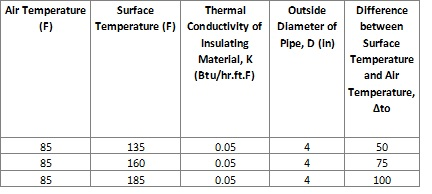2 – Find surface function (φ) from Chart 1

Based on chart below, we can get surface function.Complete the table with surface function.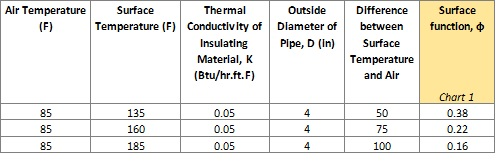3 – Find insulation thickness (X) for each surface function (φ)

Based on chart below, we get insulation thickness.Complete the table with insulation thickness.4 – Find τ1 and τ2

τ1 is a function of surface. For cylindrical vertical surface, τ1 is 1.187.

τ2 can be found easily by using Chart 1. It is a function of ∆to. See Step 2 for the procedure.

Complete the table with τ1, τ2, a, and b.5 – Calculate h

h is calculated by using equation 1. Complete the table with h.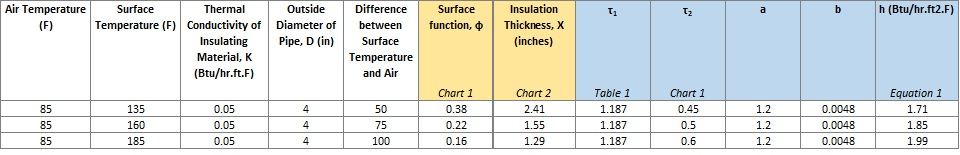6 – Calculate τ

τ is calculated by using equation 2. Complete the table with τ.7 – Find X/D (ratio of insulation thickness to pipe diameter) and X

X/D is a function of τ. Use Chart 3 to find X/D and calculate X. Complete the table.8 – Make a Plot

Plot ∆to and insulation thickness we found from Step 3 and Step 7. Connect them with two smooth curves.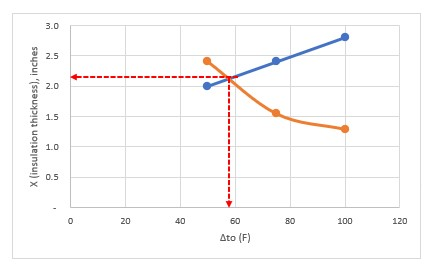From figure above, we found that at intersection of the two curves, ∆to is 58oF and optimum thickness is 2.1 inches.

References:

Evans, F. L., Equipment Design Handbook for Refineries and Chemical Plants, Vol. 1, 2nd Ed., Gulf Publishing Co., 1979.